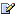CTK Exchange Front Page Movie shortcuts Personal info Awards Reciprocal links Terms of use Privacy Policy Cut The Knot! MSET99 Talk Games & Puzzles Arithmetic/Algebra Geometry Probability Eye Opener Analog Gadgets Inventor's Paradox Did you know?... Proofs Math as Language Things Impossible My Logo Math Poll Other Math sit's Guest book News sit's Recommend this siteCTK Exchange

 Subject: "Real or complex number" Previous Topic | Next Topic
 ConferencesThe CTK ExchangeHigh schoolTopic #327Printer-friendly copyEmail this topic to a friend Reading Topic #327
bitrak
Member since Jul-14-05
Jul-17-05, 02:03 PM (EST)"Real or complex number"

 What's i^i=? ; i=sqrt(-1)

Subject     Author     Message Date     IDReal or complex number bitrak Jul-17-05 TOPRE: Real or complex number alexb Jul-17-05 1RE: Real or complex number bitrak Jul-17-05 2RE: Real or complex number alexb Jul-17-05 3RE: Real or complex number JJJul-18-05 4RE: Real or complex number bitrak Jul-18-05 5RE: Real or complex number alexb Jul-18-05 6RE: Real or complex number kfomJul-06-06 7RE: Real or complex number JJJul-07-06 8RE: Real or complex number gJul-08-06 9RE: Real or complex number JJJul-09-06 10RE: Real or complex number JJJul-09-06 11

 Conferences | Forums | Topics | Previous Topic | Next Topic
alexb
Charter Member
1858 posts
Jul-17-05, 02:05 PM (EST)1. "RE: Real or complex number"
In response to message #0

 >What's i^i=? ; Just in a second, but first please tell us what do you mean by>i=sqrt(-1) What does sqrt(-1) mean in your view?bitrak
Member since Jul-14-05
Jul-17-05, 03:38 PM (EST)2. "RE: Real or complex number"
In response to message #1

 Let i^2=-1 .Calculate i^i=?alexb
Charter Member
1858 posts
Jul-17-05, 03:40 PM (EST)3. "RE: Real or complex number"
In response to message #2

 Please have a look at https://www.cut-the-knot.org/do_you_know/complex.shtmlJJguest
Jul-18-05, 07:13 AM (EST)

4. "RE: Real or complex number"
In response to message #3

 i = exp(i*pi/2)i^i = exp(i*(i*pi/2)) = exp(-pi/2)bitrak
Member since Jul-14-05
Jul-18-05, 11:38 AM (EST)5. "RE: Real or complex number"
In response to message #4

 JJ you want to say that i^i have one real solution?alexb
Charter Member
1858 posts
Jul-18-05, 11:42 AM (EST)6. "RE: Real or complex number"
In response to message #5

 >JJ you want to say that i^i have one real solution? This question I can't answer. However, do please check the link I gave earlier. There is a good discussion of this issue.

kfomguest
Jul-06-06, 08:19 PM (EST)

7. "RE: Real or complex number"
In response to message #0

 I know this sounds really bizarre... I read some book a long time ago, something like "The Story of sqrt(-1)" anyway, all I can remember is the first digit:i^i = 0.2... It's real!It's mindblowing!JJguest
Jul-07-06, 07:34 AM (EST)

8. "RE: Real or complex number"
In response to message #7

 This isn't surprising at all. Operations and/or functions involving complex numbers often leads to real number. For example, the most simplest cases are :i*i = -1i^i = exp(-pi/2)ln(i)=pi/2cos(i)=cosh(1)cosh(i)=cos(1)gguest
Jul-08-06, 11:08 PM (EST)

9. "RE: Real or complex number"
In response to message #8

 how would you start to compute i^i? t seemes so vague...JJguest
Jul-09-06, 05:47 AM (EST)

10. "RE: Real or complex number"
In response to message #9

 First : computation of real and imaginary parts of the logarithm of a complex numberln(a+i.b)=x+i.y hence a+i.b = exp(x+i.y) = exp(x).exp(i.y) = exp(x).(cos(y)+i.sin(y))a = exp(x).cos(y) and b = exp(x).sin(y)a^2 + b^2 = exp(2x) and b/a = tan(y)x = (1/2)ln(a^2 +b^2)y = atan(b/a)Case of a=0, b=1 : x=0 and y=pi/2hence : ln(i) = i.pi/2.Second : computation of i^i c^p = exp(p.ln(c)) hence i^i = exp(i.ln(i))ln(i)=i.pi/2 hence i^i = exp(i.(i.pi/2)) i^i = exp((i^2)pi/2) = exp((-1)pi/2)i^i = exp(-pi/2)

JJguest
Jul-09-06, 05:47 AM (EST)

11. "RE: Real or complex number"
In response to message #0

 Why the signs "plus" aren't correctly transmited in the equations ?

 Conferences | Forums | Topics | Previous Topic | Next Topic
 Select another forum or conference Lobby The CTK Exchange (Conference)   |--Early math (Public)   |--Middle school (Public)   |--High school (Public)   |--College math (Public)   |--This and that (Public)   |--Guest book (Public) Educational Press (Conference)   |--No Child Left Behind (Public)   |--Math Wars (Public)   |--Mathematics and general education (Public)You may be curious to have a look at the old CTK Exchange archive.